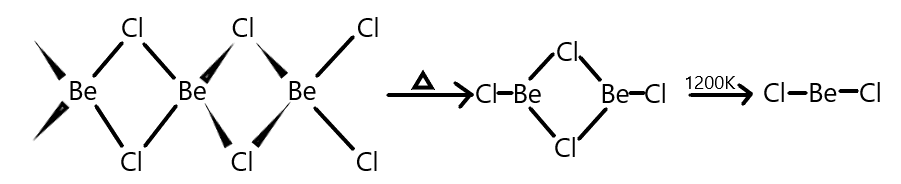QuestionAnswers

# How many p-orbital (s) is/are involved in hybridisation of solid $BeC{l_2}$?Verified
Hint:We all know that Beryllium belongs to group $2$ elements possessing an oxidation state of $+ 2$ and we are also aware with the fact that, $BeC{l_2}$ that is Beryllium chloride in vapour phase form a chloro-bridged dimer which at high temperature of the order of $1200K$ dissociates into a linear monomer.
As we know that the atomic orbitals combine to form a new set of equivalent orbitals known as the hybrid orbitals and it helps in explaining the shape of molecules and equivalency of bonds. It can be classified into $sp,s{p^2},s{p^3},s{p^3}d$ and so on which involves the number of s and p-orbitals as given in their hybridisation formula.
Let us recall that the hybridisation of linear molecules like in $BeC{l_2}$ is $sp$ hybridisation but we also know that in vapour phase $BeC{l_2}$forms a chloro-bridged dimer structure which can be converted to solid form on heating and the structure of $BeC{l_2}$then can be given as shown below:Now, as we know that Beryllium has an atomic number of $4$ and it possesses $2$ electrons in its outermost valence shell which it shares with one chlorine atom each. So, $BeC{l_2}$will have a one s and one p-orbital bonding with each chlorine atom giving it a $sp$ hybridisation. But when in solid phase, Beryllium is attached with two chlorines each where chlorine has one sigma and three lone pairs of electrons out of which only one is shared with beryllium and to form a sigma bond and rest and remaining as lone pairs. This results in the $s{p^3}$ hybridisation of the Beryllium chloride.
Therefore, from the above explanation we can say that three p-orbitals are involved in the hybridisation of solid $BeC{l_2}$.